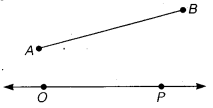# Class 6 Maths NCERT Solutions for Chapter 14 Practical Geometry Ex – 14.3

## Practical Geometry

Question 1.
Draw any line segment$\overline { PQ }$. Without measuring$\overline { PQ }$, construct a copy of$\overline { PQ }$.

Solution:
Steps of Construction:

Let PQ be the given line segment and l be a given line.1. Mark a point 0 on the line l.
2. Open out the compasses and adjust so that the steel end is on A and the pencil end is A on B.
3. Transfer the compasses to the line l without disturbing their opening so that the steel end is on 0.
4. With the pencil end make a small stroke on the line l to cut it at the point P.
Then, the fine segment OP so obtained = the given line segment AB.

Question 2.
Given some line segment$\overline { AB }$, whose length you do not know, construct$\overline { PQ }$ such that the length of$\overline { PQ }$ is twice that of$\overline { AB }$.

Solution:
Steps of Construction:

Let AB be the given line segment.Draw any line l and make a point 0 on it.

1. Open out the compasses in such way that the steel end is on A and the pencil end is on B.
2. Transfer the compasses without disturbing their opening to the line l so that the steel end is on O.
3. With the pencil end make a small stroke on -the line l to cut it at the point P.
4. Repeat the steps 3 and 4 with same opening having P as the initial point and Q as the terminal point.
Then, the segment OQ = OP + PQ = AB + AB= 2 AB.